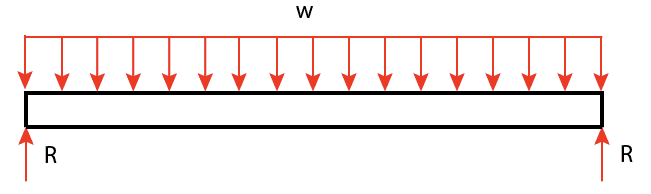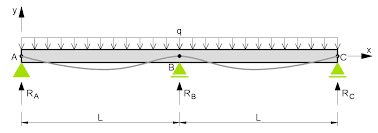# Having trouble calculating reaction forces for this truss.

• Comfy
Both beams are loaded in a similar fashion, except the latter beam requires an extra equation in addition to the equations of static equilibrium in order to solve for the reactions at the supports. In the case of the latter beam, we must know how the beam deflects under load, and we cannot calculate that unless we know some additional structural details beyond the spacing of the supports.

## Homework Statement

Calculate reaction forces for truss. Truss is attached in picture.

ΣFx=o
ΣFy=0

## The Attempt at a Solution

attached in picture

Comfy said:

## Homework Statement

Calculate reaction forces for truss. Truss is attached in picture.

ΣFx=o
ΣFy=0

## The Attempt at a Solution

attached in picture
Unfortunately, the attached images are almost entirely illegible.If you want some help, either write out your calculations or post better images.SteamKing said:
Unfortunately, the attached images are almost entirely illegible.If you want some help, either write out your calculations or post better images.This truss is statically determinate now, and should be solvable, right? That is why I don't understand why I am having trouble solving for the support reactions.

#### Attachments

Comfy said:
This truss is statically determinate now, and should be solvable, right? That is why I don't understand why I am having trouble solving for the support reactions.

What makes you think this truss is statically determinate? You're still trying to calculate four reactions using only two equations of statics.

SteamKing said:
What makes you think this truss is statically determinate? You're still trying to calculate four reactions using only two equations of statics.
I
SteamKing said:
What makes you think this
SteamKing said:
What makes you think this truss is statically determinate? You're still trying to calculate four reactions using only two equations of statics.
I have more than 2 equations, I have sum of forces in y and sum of moments about any of the supports. It is statically determinate because 2 (11 joints)=17 members+5 reactions. What do you mean by 2 equations of statics?
truss is statically determinate? You're still trying to calculate four reactions using only two equations of statics.
I have 4 equations Sum of forces in y direction and the

Comfy said:
I

I have 4 equations Sum of forces in y direction and the
Your last post came out garbled. Can you repeat?

SteamKing said:
Your last post came out garbled. Can you repeat?
I have more than 2 equations, I have sum of forces in y and sum of moments about any of the supports. It is statically determinate because 2 (11 joints)=17 members+5 reactions. What do you mean by 2 equations of statics?

Comfy said:
I have more than 2 equations, I have sum of forces in y and sum of moments about any of the supports. It is statically determinate because 2 (11 joints)=17 members+5 reactions. What do you mean by 2 equations of statics?
You can write only one equation involving the sum of the forces and one equation summing the moments about one convenient reference point.

You cannot write, for example, one moment equation where you sum moments about point A and another equation where you sum moments about point H.

This beam is statically determinate:This beam is statically indeterminate:Both beams are loaded in a similar fashion, except the latter beam requires an extra equation in addition to the equations of static equilibrium in order to solve for the reactions at the supports. In the case of the latter beam, we must know how the beam deflects under load, and we cannot calculate that unless we know some additional structural details beyond the spacing of the supports.